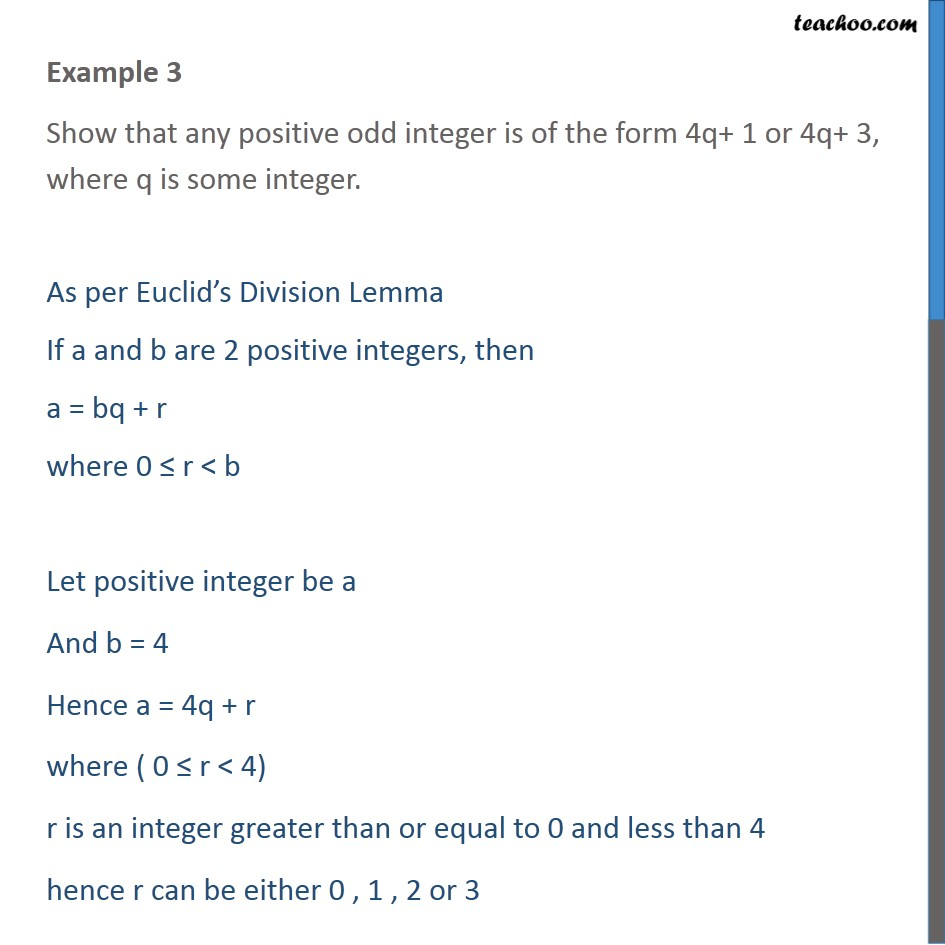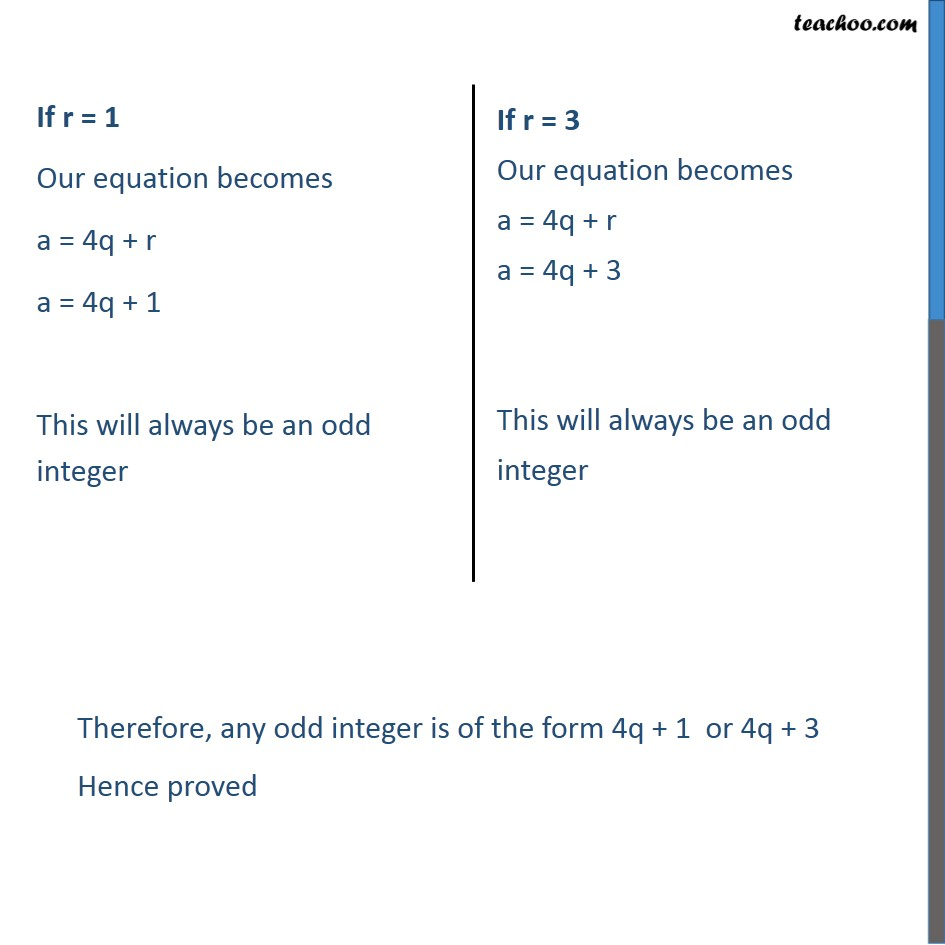Check Important Questions and Chapter Summary -https://you.tube/Real-Numbers-Class-101. Chapter 1 Class 10 Real Numbers
2. Concept wise
3. Euclid's Division Algorithm - Proving

Transcript

Example 3 Show that any positive odd integer is of the form 4q+ 1 or 4q+ 3, where q is some integer. As per Euclid s Division Lemma If a and b are 2 positive integers, then a = bq + r where 0 r < b Let positive integer be a And b = 4 Hence a = 4q + r where ( 0 r < 4) r is an integer greater than or equal to 0 and less than 4 hence r can be either 0 , 1 , 2 or 3

Euclid's Division Algorithm - Proving